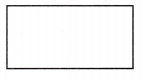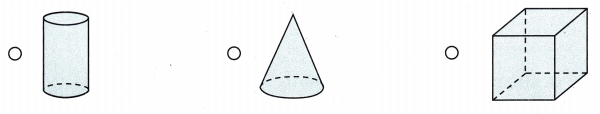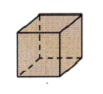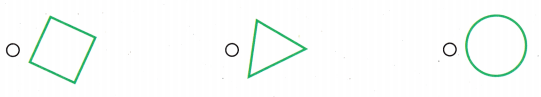# Texas Go Math Grade 1 Lesson 15.1 Answer Key Two-Dimensional Shapes on Three-Dimensional Solids

Refer to our Texas Go Math Grade 1 Answer Key Pdf to score good marks in the exams. Test yourself by practicing the problems from Texas Go Math Grade 1 Lesson 15.1 Answer Key Two-Dimensional Shapes on Three-Dimensional Solids.

## Texas Go Math Grade 1 Lesson 15.1 Answer Key Two-Dimensional Shapes on Three-Dimensional Solids

Explore

Use a cone.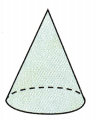FOR THE TEACHER • Read the following problem, and have children use the workspace to act it out. Lee places a cone on a piece of paper and draws around its flat surface. What does Lee draw?
Answer: Lee draws a flat surface with the help of a cone

Math Talk
Mathematical Processes

What other solid could you use to draw the same kind of picture? Explain.
Answer: A cylinder also has two flat surfaces, so the other solid object can be used is a cylinder to draw a flat surface like the cone.Model and Draw

Trace around the flat surfaces of the three-dimensional solid to find the two-dimensional shapes.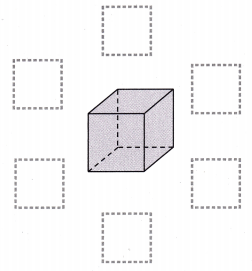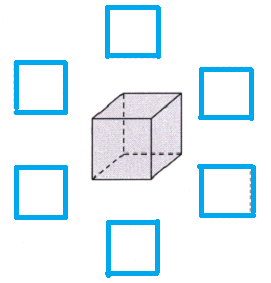Share and Show

Use three-dimensional solids. Trace around the flat surfaces. Circle the shapes you draw.

Question 1.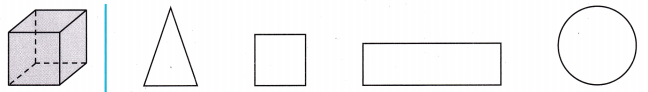Question 2.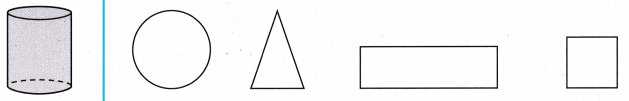Question 3.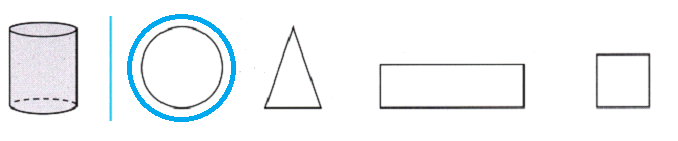Problem Solving

Circle the objects you could trace to draw the shape.

Question 4.Question 5.Question 6.
H.O.T. Circle the solid that the pattern will make if you fold it and tape it together.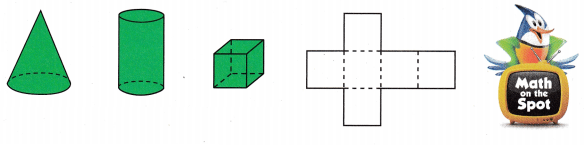Answer: Cube will be formed if we fold the pattern and tape it together.

Question 7.
H.O.T. Multi-Step Draw two different shapes you could make if you traced this solid.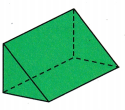Tracing triangular prism we can draw 2 different shapes which are a triangle and a rectangle.

Question 8.
Carl buys a toy for his cat. The top and the bottom of the toy looks like this. Which shows the shape of the toy?In cylinder shape both top and bottom is having flat surfaces, Carls buys a cylidrical shaped toy for his cat.

Question 9.
Analyze Donna buys a toy for her dog. Only the bottom of the toy looks like this. Which shows the shape of the toy?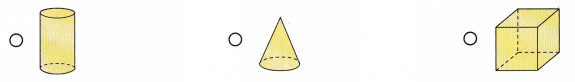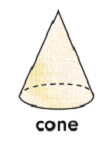Cone is having only one flat surface, Donna buys a cone shaped toy for her dog.

Question 10.
Multi-Step Judy traces two sides of a solid. Draw the solid that she uses. Explain how you know that your answer is reasonable.Question 11.
Texas Test Prep Which flat surface does a cube have?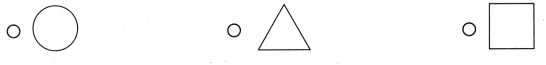Answer: Cube has square shaped flat surface

TAKE HOME ACTIVITY • Collect a few three-dimensional objects that are shaped like spheres, cones, cylinders, and cubes. Ask your child what two-dimensional shapes are on those objects.
Answer: Three dimensional objects that are shaped like: sphere, cone, cylinder and cube.

### Texas Go Math Grade 1 Lesson 15.1 Homework and Practice Answer Key

Look at the three-dimensional solid. Circle the shapes you would draw if you traced around the flat surfaces.

Question 1.Cube has a flat squared surfaces.

Question 2.Cylinder has 2 flat surfaces, one at the top and second at the bottom.

Problem Solving

Question 3.
Draw a shape you could make if you traced this cube.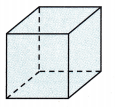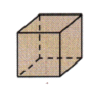Lesson Check

Question 4.
Maggie bakes a cake. The top and the bottom of the cake look like this. Which shows the shape of the cake?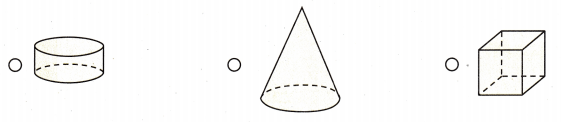Cake has flat surface both at the top and bottom.

Question 5.
Multi-Step Michael makes a board game in the shape of a rectangle. He traces around two faces of a solid. Which solid does he trace?